Function Repository Resource:

# TotalSignedCurvature

Compute the total signed curvature of a curve

Contributed by: Wolfram Staff (original content by Alfred Gray)
 ResourceFunction["TotalSignedCurvature"][c,t] computes the total signed curvature of a curve c with respect to parameter t. ResourceFunction["TotalSignedCurvature"][c,{t,a,b}] computes the total signed curvature from a to b.

## Details and Options

The signed curvature κ of a plane curve c is defined as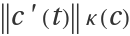, and measures the bending of the curve at each of its points. A measure of the total bending of c is given by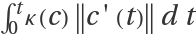.

## Examples

### Basic Examples (4)

Compute the total signed curvature of a circle:

 In:=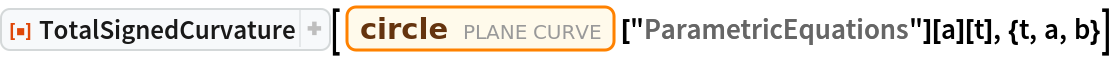Out=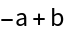Some results are very similar:

 In:=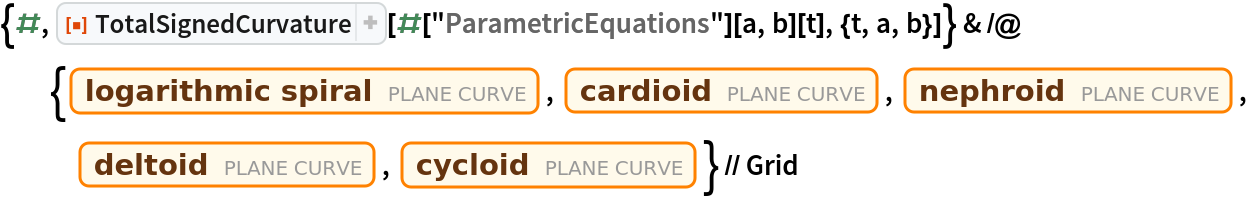Out=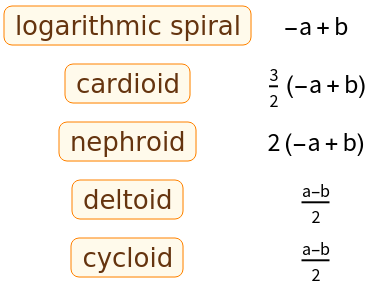Some results involve inverse functions:

 In:=Out=Find the result for a Cornu spiral:

 In:=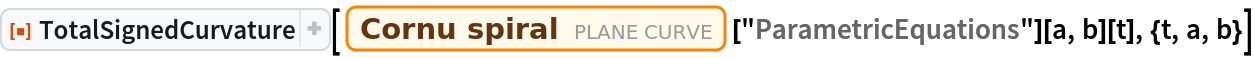Out=### Properties and Relations (4)

Define a hypotrochoid function:

 In:=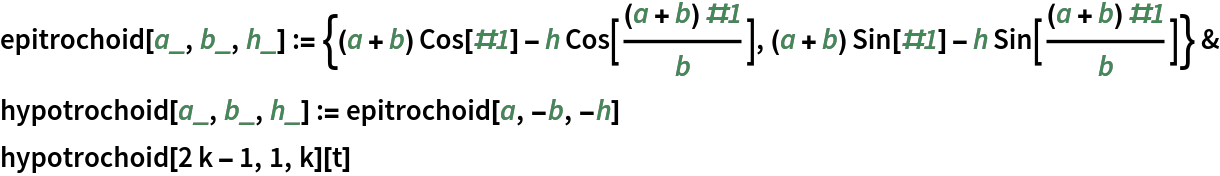Out=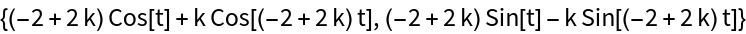The total signed curvature of a hypotrochoid:

 In:=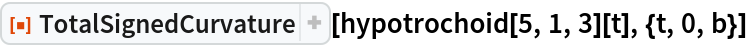Out=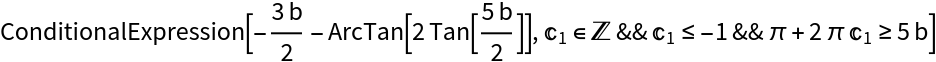Plots of several hypotrochoids:

 In:=Out=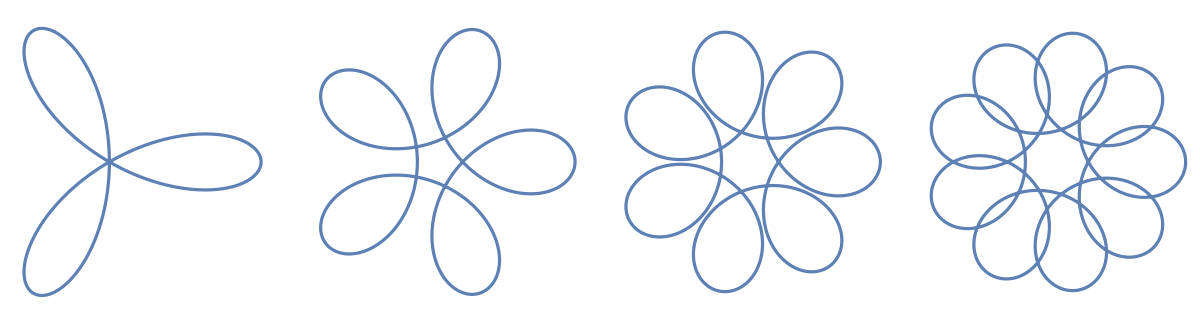The total signed curvature is related to turning numbers:

 In:=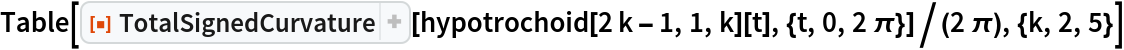Out=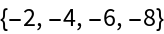In:=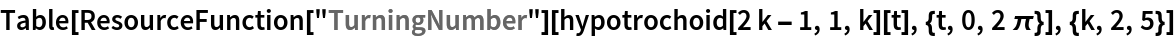Out=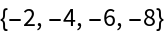Enrique Zeleny

## Version History

• 1.0.0 – 14 August 2020